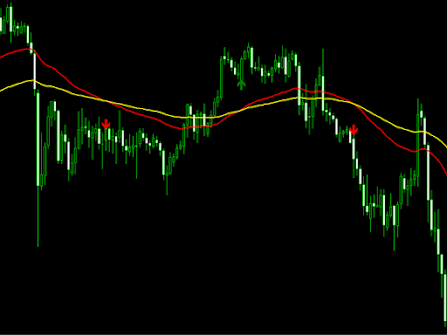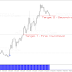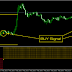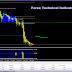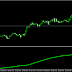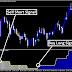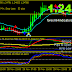-->

# Moving Average [MA] Crossover Arrows Indicator for MT4 and MT5 | 100% FREE TO DOWNLOAD

Moving averages are commonly implemented in technical analysis, a subset of investing that aims to comprehend and profit from the price movement patterns of stocks and indices. Moving averages are commonly used by technical analysts to spot a shift in momentum for an asset, such as a sudden downward move in a security's price. At times, they will employ moving averages to corroborate their concerns that a shift is taking place. For example, a company's share price rising above its 200-day moving average could be interpreted as a bullish indicator.

Moving Average [MA] Crossover Arrows is a simple indicator that draws arrows and alerts when two moving averages crossover.

Supported types of Moving Averages

• Simple
• Exponential
• Smoothed
• Linear Weighted

Supported Price types

• Close
• Open
• High
• Low
• Median Price (HL/2)
• Typical (HLC/3)
• Weighted Close (HLCC/4)
When the fast moving average crosses above the slow moving average, a green up arrow will appear on the chart. When the fast moving average crosses below the slow moving average, a red down arrow will appear on the chart.

Indicator options and settings
Settings for fast moving average

• FastMA = Averaging period of fast moving average.
• FastMAType = Moving average method for the fast moving average.
• Fast_Applyto = Price type used for calculating fast moving average.
• Settings for slow moving average

• SlowMA = Averaging Period of slow moving average.
• SlowMAType = Moving average method for slow moving average.
• Slow_Applyto = Price type used for calculating slow moving average.